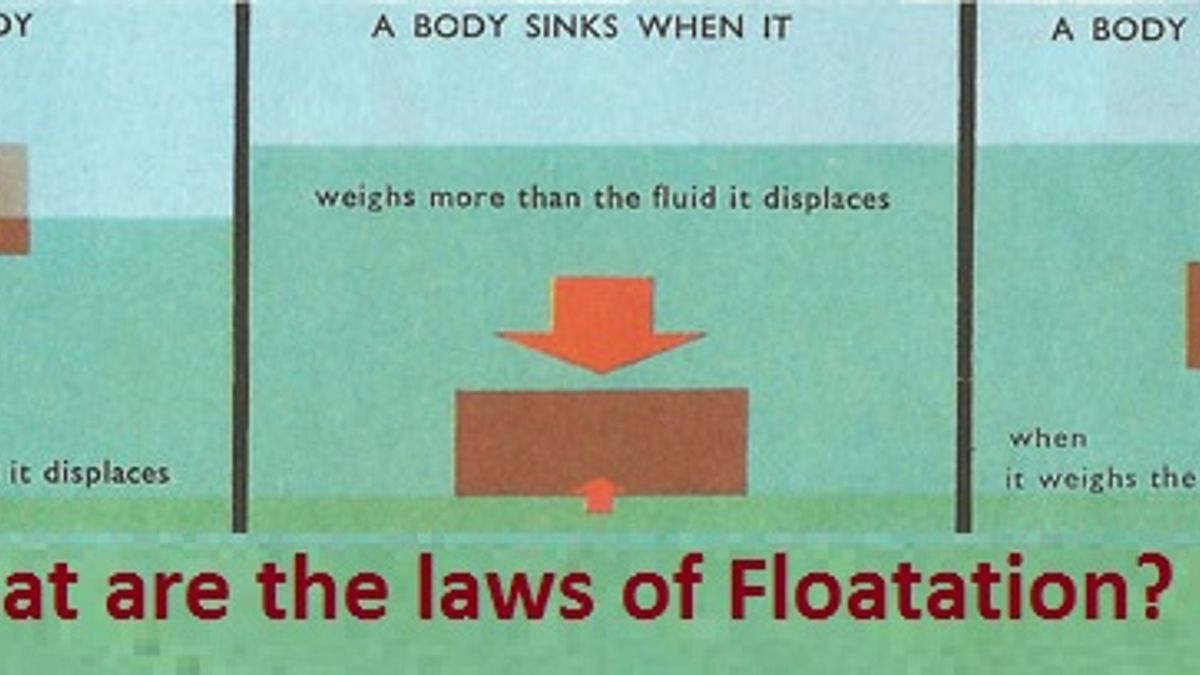# What are the laws of Floatation?

Floatation depends upon the density. If an object has density less than the density of water, it floats. Principle of floatation is stated by the Archimedes. This article deals with what is floatation, laws of floatation, its applications and examples.State the Laws of Floatation

Archimedes, the Ancient Greek scientist first stated the principle of floatation. According to him, all the objects placed in a liquid experience an upward force which allows the body to float if it displaces water with the weight equal to the weight of the body. This upward force is known as buoyant force and the law is known as the law of buoyancy. Mainly, floatation depends upon the density. If an object has a density less than the density of water, it floats. Like, leaf of a plant floats on the water because the density of leaf is less than the density of water. A stone thrown in water sinks because the density of stone is more than the density of water.
Have you ever thought that a ship weighing several tons floats while a needle sinks? This can be explained as follows: A ship is made up of iron and steel, but it has a lot of space filled with air. This causes the ship to displace water with a weight equal to the weight of the ship. On the other hand, the needle displaces more water than its weight and hence it sinks.
What is Archimedes Principle?

Source: www.image.slidesharecdn.com
When a body is immersed partly or wholly in a liquid, there is an apparent loss in the weight of the body which is equal to the weight of liquid displaced by the body.

What is the reason behind floating ice on water
Laws of Floatation
A body floats in a liquid if:
1. Density of the material of the body is less than or equal to the density of the liquid.
2. If density of material of body is equal to density of liquid, the body floats fully submerged in liquid in neutral equilibrium.
3. When body floats in neutral equilibrium, the weight of the body is equal to the weight of displaced liquid.
4. The centre of gravity of the body and centre of gravity of the displaced liquid should be in one vertical line.
Now, we will understand Centre of Buoyancy and Meta Centre
Centre of Buoyancy: The centre of gravity of the liquid displaced by a body is known as centre of buoyancy.
Meta centre: When a floating body is slightly tilted from equilibrium position, the centre of buoyancy shifts. The point at which the vertical line passing through the new position of centre of buoyancy meets with the initial line is called meta centre.
What are the conditions for stable equilibrium for floating body?
a. The meta centre must always be higher than the centre gravity of the body.
b. The line joining the centre of gravity of the body and centre of floatation should be vertical.
We know density is mass per unit volume. Its S.I unit is kg/m3 and relative density is density of material per density of water at four degree Celsius. Relative density is measured by Hydrometer.
- The density of sea water is more than that of normal water. That is why it is easier to swim in sea water.
- When ice floats in water, its 1/10th the part remain outside the water.
- If ice floating in water in a vessel melts, the level of water in the vessel does not change.
- Purity of milk is measured by lactometer.
From the article we have learnt that what is floatation, how can we swim, why some objects floats instead of sinking, what are the laws of floatation etc.

Do you know why Oil and Water does not mix together?

Get the latest General Knowledge and Current Affairs from all over India and world for all competitive exams.
खेलें हर किस्म के रोमांच से भरपूर गेम्स सिर्फ़ जागरण प्ले पर